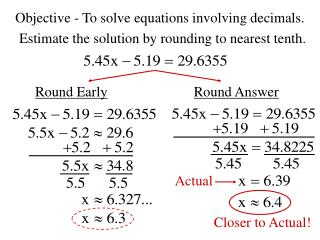DownloadDownload PresentationObjective - To solve equations involving decimals.

# Objective - To solve equations involving decimals.

Télécharger la présentation## Objective - To solve equations involving decimals.

- - - - - - - - - - - - - - - - - - - - - - - - - - - E N D - - - - - - - - - - - - - - - - - - - - - - - - - - -
##### Presentation Transcript

1. Objective - To solve equations involving decimals. Estimate the solution by rounding to nearest tenth. Round Early Round Answer Actual Closer to Actual!

2. Solve. Round to the nearest tenth.

3. Solve. Round to the nearest tenth.

4. Solve. Round to the nearest hundredth.

5. Solve. Round to the nearest hundredth.

6. Solve using a variable equation. Yellow Cab charges an initial \$3.75 and 32 cents per mile. Checker Taxi charges \$6.50 to begin a fare and 26 cents per mile. After how may miles will both fares be equal? Let x = # of miles driven Yellow Cab Checker Taxi

7. Solve using a variable equation. At&T charges a monthly service fee of \$15.80 and 8 cents per minute. Sprint charges \$23.50 initially but only 5 cents per minute. After how many minutes do they charge the same? Let n = # of minutes At&T Sprint